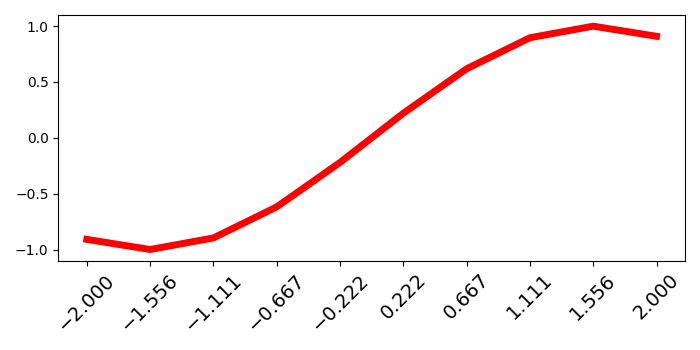# How can I change the font size of ticks of axes object in Matplotlib?

To change the font size of ticks of axes object in matplotlib, we can take the following steps −

• Create x and y data points using numpy.

• Using subplots() method, create a figure and a set of subplots (fig and ax).

• Plot x and y data points using plot() method, with color=red and linewidth=5.

• Set xticks with x data points.

• Get the list of major ticks using get_major_ticks() method.

• Iterate the major ticks (from step 5), and set the font size and rotate them by 45 degrees.

• To display the figure, use show() method.

## Example

import numpy as np
from matplotlib import pyplot as plt
plt.rcParams["figure.figsize"] = [7.00, 3.50]
plt.rcParams["figure.autolayout"] = True
x = np.linspace(-2, 2, 10)
y = np.sin(x)

fig, ax = plt.subplots()

ax.plot(x, y, c='red', lw=5)
ax.set_xticks(x)
for tick in ax.xaxis.get_major_ticks():
tick.label.set_fontsize(14)
tick.label.set_rotation('45')

plt.tight_layout()

plt.show()

## Output